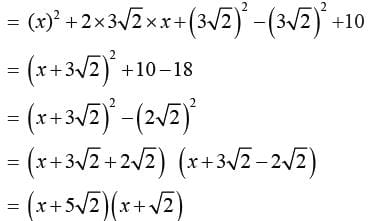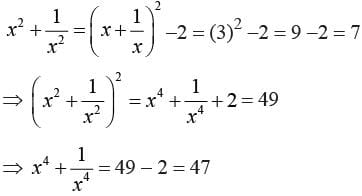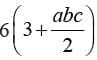Math Olympiad Test: Number System- 2

# Math Olympiad Test: Number System- 2

Test Description

## 15 Questions MCQ Test Mathematics Olympiad for Class 9 | Math Olympiad Test: Number System- 2

Math Olympiad Test: Number System- 2 for Class 9 2022 is part of Mathematics Olympiad for Class 9 preparation. The Math Olympiad Test: Number System- 2 questions and answers have been prepared according to the Class 9 exam syllabus.The Math Olympiad Test: Number System- 2 MCQs are made for Class 9 2022 Exam. Find important definitions, questions, notes, meanings, examples, exercises, MCQs and online tests for Math Olympiad Test: Number System- 2 below.
Solutions of Math Olympiad Test: Number System- 2 questions in English are available as part of our Mathematics Olympiad for Class 9 for Class 9 & Math Olympiad Test: Number System- 2 solutions in Hindi for Mathematics Olympiad for Class 9 course. Download more important topics, notes, lectures and mock test series for Class 9 Exam by signing up for free. Attempt Math Olympiad Test: Number System- 2 | 15 questions in 15 minutes | Mock test for Class 9 preparation | Free important questions MCQ to study Mathematics Olympiad for Class 9 for Class 9 Exam | Download free PDF with solutions
 1 Crore+ students have signed up on EduRev. Have you?
Math Olympiad Test: Number System- 2 - Question 1

### If x - 3 is a factor of αx2 + 18 = 0, then a =

Detailed Solution for Math Olympiad Test: Number System- 2 - Question 1

α - (-3) is a factor of f(x)
∴ f(-3) = 0
⇒ α (-3)2 + 18 = 0
⇒ α = -2

Math Olympiad Test: Number System- 2 - Question 2

### If  x - 1 is a factor of 4x3 + 3x2 - 4x + k , then k =

Detailed Solution for Math Olympiad Test: Number System- 2 - Question 2

If x = 1 is a factor of f(x), then f(1) = 0
⇒ = f(1) = 4 (1)3 + 3(1)2 - 4 (1) + k = 0
= 4 + 3 - 4 + k = 0
⇒ k = -3

Math Olympiad Test: Number System- 2 - Question 3

### Factors of x2 + 6√2x + 10 will be

Detailed Solution for Math Olympiad Test: Number System- 2 - Question 3

Here x2 + 6√2x + 10Math Olympiad Test: Number System- 2 - Question 4

If  x + 1/x = 3 then x4 + 1/x4

Detailed Solution for Math Olympiad Test: Number System- 2 - Question 4

Given x + 1/x = 3Math Olympiad Test: Number System- 2 - Question 5

If α = b = c, then (a + b + c)2 - xα2 = 0, then x =

Detailed Solution for Math Olympiad Test: Number System- 2 - Question 5

If α = b = c, then
(α + b + c) = (α + α + α) = 3α
∴ (a + b + c)2 - xα2 = (3α)2 - xα2 = 0
⇒ xα2 = 9α2
⇒ x = 9

Math Olympiad Test: Number System- 2 - Question 6

The value of 9α2 +  4b2 + 16c2 + 12αb - 24ac -16bc for b = 1, c = - 2 will be

Detailed Solution for Math Olympiad Test: Number System- 2 - Question 6

2 +  4b2 + 16c2 + 12αb - 24ac -16bc
= (3α)2 + (2b)2 + (- 4c)2 + 2 (3α)(2b) + 2(3α)(-4c) + 2 (3a)(-4c)
= (3a + 2b - 4c)2
Putting α = 2, b = 1, c = -2, we have required expression as
[3(2) + 2 (1) - 4 (-2)]2
= [ 6 + 2 + 8]2 = (16)2 = 256

Math Olympiad Test: Number System- 2 - Question 7

If 3x + 2y = 13 and xy = 6, then 27x3 + 8y3 will be equal to

Detailed Solution for Math Olympiad Test: Number System- 2 - Question 7

Given 3x + 2y = 13, xy = 6
∵ 3x + 2y = 13
∴ (3x + 2y)3 = (13)3
⇒ 27x3 + 8y3 + 3 × 3x × 2y (3x + 2y)
= (169) × 13
⇒ 27x3 + 8y3 + 18xy (3x + 2y) = (169) × 13
⇒ 27x3 + 8y3 + 18 × 6 × (13) = (169) × 13
⇒ 27x3 + 8y3 = 61 × 13 = 793

Math Olympiad Test: Number System- 2 - Question 8

The value of (x - 1) (x2 + 1 + x) (x6 + x3 + 1), will be equal to

Detailed Solution for Math Olympiad Test: Number System- 2 - Question 8

We have (x - 1) (x2 + x + 1) = (x3 - 1)
Now,
Let x3 = p, then x6 = p2
∵ (x3 - 1) (x6 + x3 + 1)
= (p - 1)(p2 + p + 1) = (p3 - 1)
= (x3)- 1
= x9 - 1

Math Olympiad Test: Number System- 2 - Question 9

(x2 - x + 1) is a factor of:

Detailed Solution for Math Olympiad Test: Number System- 2 - Question 9

(x4 +  x2 + 1) has two factors,
i.e, (x2 +  x + 1) and (x2 -  x + 1)

Math Olympiad Test: Number System- 2 - Question 10

If x + y = 4, xy = 4, then 2x3 + y3 will be equal to

Detailed Solution for Math Olympiad Test: Number System- 2 - Question 10

Given x + y = 4, xy = 4
⇒ (x + y)2 = (x - y)2 + 4xy
⇒ (4)2 = (x - y)2 + 4 × 4
⇒ (x - y)2 = 16 - 16 = 0
⇒ x = y
∴ if x + y = 4
⇒ y = x = 2
Hence, 2x3 + y3 = 3x3 = 3(2)3 = 24

Math Olympiad Test: Number System- 2 - Question 11

If the polynomials αx3 + 4x2 + 3x - 4 and x- 4x + α equal to

Detailed Solution for Math Olympiad Test: Number System- 2 - Question 11

f(3) will be remained in both cases.
∴ α(3)3 + 4(3)3 + 3(3) - 4 = (3)3 - 4(3) + α
⇒ 27α + 36 + 9 - 4 = 27 - 12 + a
⇒ 26α = 15 - 5 - 36
⇒ 26α = -26
⇒ α = -1

Math Olympiad Test: Number System- 2 - Question 12

If α + b + c = 15, and α2 + b2 + c2 = 83, then the value of α3 + b+ c3 - 3αbc will be

Detailed Solution for Math Olympiad Test: Number System- 2 - Question 12

α3 + b3 + c3 - 3αbc = (α + b + c) (α2 + b2 + c2 - αb - bc - cα) = (15) (83 - 71)
= 15 (12)
= 180

Math Olympiad Test: Number System- 2 - Question 13

If α + b + c = 15 and α2 + b2 + c2 = 83 then the value of (αb + bc + cα) will be equal to

Detailed Solution for Math Olympiad Test: Number System- 2 - Question 13

Here (α + b + c)2 = (15)2
⇒ (α2 + b2 + c2) = (15)2 - 2(αb + bc + cα)
⇒ (83) - 225/2 = - (αb + bc + cα)
⇒ - 71 = - (αb + bc + cα) ...(i)
⇒ αb + bc + cα = 71 ...(i)

Math Olympiad Test: Number System- 2 - Question 14

The expression (α - b)3 + (b - c)3 + (c - α)3 can be factorised as

Detailed Solution for Math Olympiad Test: Number System- 2 - Question 14

Let α - b = A, b - c = B,  c - α = C
Then, A + B + C = (α - b) + (b - c) + (c - α) = 0
∴ A3 + B3 + C3 = 3ABC
⇒ 3(α - b) (b - c) (c - α)

Math Olympiad Test: Number System- 2 - Question 15

If α + b + c = 6 and α3 + b+ c3 =, then αb + bc + cα will have the value equal to

Detailed Solution for Math Olympiad Test: Number System- 2 - Question 15

Given α + b + c = 6 , and, α3 + b3 + c3 = 18 + 3αbc
⇒ a3 + b3 + c3 - 3αbc = 18

## Mathematics Olympiad for Class 9

1 videos|43 tests
 Use Code STAYHOME200 and get INR 200 additional OFF Use Coupon Code
Information about Math Olympiad Test: Number System- 2 Page
In this test you can find the Exam questions for Math Olympiad Test: Number System- 2 solved & explained in the simplest way possible. Besides giving Questions and answers for Math Olympiad Test: Number System- 2, EduRev gives you an ample number of Online tests for practice

## Mathematics Olympiad for Class 9

1 videos|43 tests